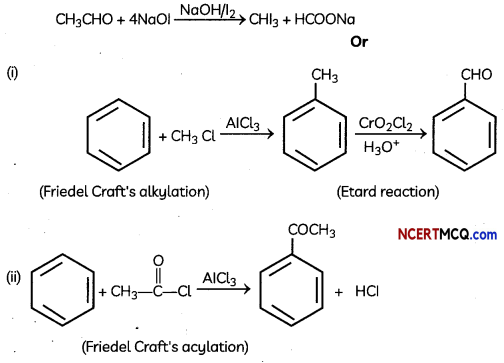Students can access the CBSE Sample Papers for Class 12 Chemistry with Solutions and marking scheme Term 2 Set 9 will help students in understanding the difficulty level of the exam.

## CBSE Sample Papersn for Class 12 Chemistry Term 2 Set 9 with Solutions

Time Allowed: 2 Hours
Maximum Marks: 35

General Instructions:

• There are 12 questions in this question paper with internal choice.
• SECTION A – Q. No. 1 to 3 are very short answer questions carrying 2 marks each.
• SECTION B – Q. No. 4 to 11 are short answer questions carrying 3 marks each.
• SECTION C- Q. No. 12 is case based question carrying 5 marks.
• All questions are compulsory.
• Use of log tables and calculators is not allowed.

Section – A
(Section A-Question No 1 to 3 are very short answer questions carrying 2 marks each.)

Question 1.
Explain what is observed:
(A) When a beam of light is passed through a colloidal solution.
When a beam of light is passed through a colloidal solution, the path of the beam gets illuminated by bluish light and this is known as Tyndall effect.

(B) Electric current is passed through solution. (2)
When the electric current is passed through colloidal solution movement of the colloidal particles either towards the cathode or towards the anode takes place. This is known as electrophoresis. Explanation: The Tyndall effect is the phenomenon in which the particles in a colloid scatter the beams of light that are directed at them. This effect is exhibited by all colloidal solutions and some very fine suspensions. Thus the identity of the colloidal solutions is determined by this effect. The density of the colloidal particles as well as the frequency of the incident light determines the intensity of the colloidal solutions.Question 2.Observe the above graph and explain the variation of conductivity with concentration for strong and weak electrolyte. (2)
Variation of conductivity depends on the concentration of the electrolyte. The conductivity and Molar conductivity of both weak and strong electrolytes decreases with decrease in concentration as the number of ions per unit volume carrying the current in a solution decreases on dilution.

For strong electrolytes number of ions per unit volume are high as they completely dissociate in solution. With dilution the interionic forces decrease therefore ionic mobility increases.
For weak electrolytes extent of dissociation is very small and with dilution the dissociation increases slowly but the value of molar conductivity at infinite dilution cannot be obtained as in the case of strong electrolyte.

Question 3.
Carry out the following conversions: (Any two)
(A) Methyl chloride to Acetic acid(B) Benzene to benzoic acid(C) Ethanol to Acetone. (2)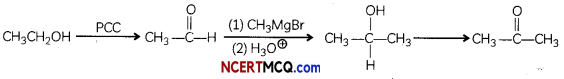Section – B
(Section B-Question No 4 to 11 are short answer questions carrying 3 marks each.)

Question 4.
(A) Calculate the rate of the reaction for the rate law -d[A]/ dt = K [A].[B]2, when the concentration of A and B are 0.1 M and 0.02 M respectively and K = 5.1 × 10-3 L2 s-1 mol-2.
(B) What is the order of the reaction for the graph given below, What does the slope depict for a graph between In [A] and time. (3)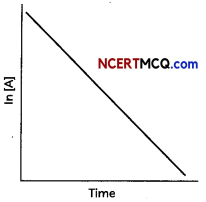(A) Given that
Rate of reaction
$$\frac{\mathrm{d}[\mathrm{A}]}{\mathrm{dt}}$$ = K [A], [B]2

Given K = 5.1 × 10-3 L2s-1 mol-1
[A] = 0.1 M, [B] = 0.02 M, then
– $$\frac{\mathrm{d} \mathrm{A}}{\mathrm{dt}}$$ = 5.1 × 10-3 × 0.1 × (0.02)2
= 0.00204 × 10-3
= 2.04 × 10-7 Ms-1

(B) For first-order reactions, the equation ln[A] = -kt + ln[A]0 is similar to that of a straight line. (y = mx + c) with slope -k. Thus, the graph for ln[A] v/s t for a first-order reaction is a straight line with slope -k

Question 5.
(A) Differentiate between physical and Chemical Adsorption.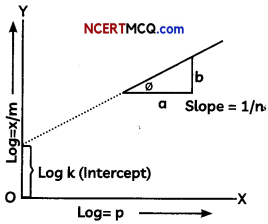OR
Give reason for the following:
(A) Finely divided substances are more effective as adsorbents.
(B) Lyophilic colloids are also called reversible sols.
(C) Agl sol, formed after AgNO3 reacts with Kl, is negatively charged. (3)
(A)

 Physical Adsorption Chemical Adsorption 1. Vander Waals force acts between adsorbate and adsorbent 1. Chemical force acts between adsorbate and adsorbent 2. It is reversible in nature. 2. It is irreversible in nature. 3. It is not specific 3. It is highly specific 4. Requires low activation energy 4. Requires high activation energy 5. Multilayers of adsorbed parti­cles are formed 5. Monolayer of adsorbed parti­cles is formed 6. It has low heat of adsorption (20 to 40 kJ/ mol). 6. It has high heat of adsorption (40 to 200 kJ/ mol).

(B) The Freundlich equation or Freundlich adsorption isotherm is shown in the graph and adsorption isotherm, is an empirical relation between the concentration of a solute on the surface of an adsorbent to the concentration of the solute in the liquid with which it is in contact.
OR
(A) The finely divided substance is more effective as an absorbent because more the surface area more is the extent of adsorption. So they act as good adsorbents.

(B) Lyophilic colloids are also called reversible sols because once separated these can be reformed by simple mixing. Thus due to this reversible property they are also regarded as reversibel sols.

(C) When silver nitrate (AgNO3) solution is added to Kl solution, a negatively charged sol is formed. It is due to the preferential adsorption of I ions and thus the Agl sol formed is negatively charged in nature.

Question 6.
(A) Give any two differences between double salts and complexes?
(B) Explain how [FeF6]3- is paramagnetic outer orbital complex. (3)
(A) The difference between Double salt and Complex compound are:

 Double Salt Complex Compound 1. These exit only in solid state and dis­sociate into constituent species in their solution. 1. They retain their identity in solid as well as in solution state. 2.  lose their identity in dissolved state. 2. They do not lose their identity in dissolved state.

(B) In [FeF6]3-
Oxidation number of Fe = +3
Coordination Number Fe = 6
Coordination orbitals = 6
Fe → Fe3+ + 3e
Fe3+ → (26-3) – [Ar]18
[FeF6]3- – (Strongly Paramagnetic)Here F is a weak field ligand and it obeys Hund’s rule of leaving maximum number of unpaired electrons (n = 5) and it uses one 4s, three 4p and two 4d orbitals which are sp3 d2 hybridised. So it is an outer orbital complex.

Question 7.
(A) What is the role of pyridine in the acylation reaction of amines ?
(B) Why is NH2 group of aniline acetylated before carrying out nitration? Explain with the help of reactions.
OR
Predict the product for the reaction of:
(A) Aniline with bromine in non-polar solvent such as CS2.
(B) C6H5CH2NH2 reacts with HNO2
(C) Reduction of nitriles with LiAlH4 (3)
(A) Pyridine and other bases are used to remove the side product i.e., HCl formed during reaction mixture.

(B) Direct nitration of aniline is not possible on account of oxidation of —NH2 group. However, nitration can be carried after protecting the —NH2 group by acetylation to give acetanilide which is then nitrated and finally hydrolysed to give o-and p-nitroanilines.

The acetyl group being electron withdrawing attracts the lone pair of electrons of the N-atom towards carbonyl group.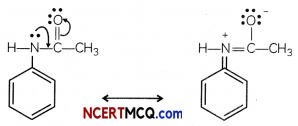As a result the activating effect —NH2 group is reduced i.e., the lone pair of electrons on nitrogen is less available for donation to because to benzene ring by resonance. Therefore, activating effect of the —NHCOCH3 group is less than that of the — NH2 group.
OR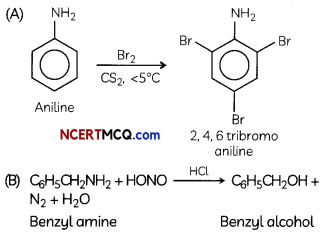(C) Reduction of nitriles with sodium alcohol or LiAlH4 gives primary amine RNH2Question 8.
(A) Decide giving reason which one of the following pairs exhibits the property indicated:
(i) Sc3+ or Cr3+ (exhibits paramagnetism)
(ii) V or Mn (exhibits more number of oxidation states)
(iii) V4+ or V5+ (exhibits colour) (3)
(A) Cr3+ exhibits parai4iognetism due to the presence of 3 unpaired electrons in 3d orbitals

Explanation:
Electronic configuration
Sc3+ : [Ar] 3d° 4s° – No unpaired electron
Cr3+ : [Ar] 3d3 4s° – Three unpaired electrons

(B) Mn shows maximum oxidation states (+2 to +7)
Explanation:
Electronic configuration:
V : [Ar] 3d3 4s2 (5 electrons can participate in bonding)
Mn : [Ar] 3d5 4s2 (7 electrons can participate in bonding)

(C) V4+ shows colour due to the presence of one unpaired electron in 3d orbital which undergoes d-d transition. V5+ does not have any unpaired electron.Question 9.
Account for the following observations:
(A) pkb value for aniline is more than that for methylamine.
(B) Methylamine in water reacts with ferric chloride to precipitate ferric hydroxide
(C) Aniline does not undergo Friedel-Craft’s reaction. (3)
(A) Aniline undergoes resonance and as a result, the electrons on the N-atom are delocalized over the benzene ring. Therefore, the electrons on the N-atom are less available to donate. In case of methylamine (due to the +1 effect of methyl group), the electron density on the N-atom is increased. As a result, aniline is less basic than methylamine. Thus, pKb of aniline is more than that of methylamine.

(B) Methylamine in water reacts with ferric chloride to precipitate hydrated ferric oxide. Due to the +1 effect of -CH3 group, methylamine is more basic than water. Therefore, in water, methylamine produces OH- ions by accepting H+ ions from water. OH” ions react with ferric chloride to precipitate hydrated ferric oxide.

(C) Aniline does not undergo Friedel Craft’s reactions because the reagent AlCl3 (a Lewis acid which is used as a catalyst in Friedel Crafts reaction), being electron deficient acts as a Lewis base, and attacks on the Lone pair of nitrogen present in aniline to form an insoluble complex which precipitates out and the reaction does not proceed.

Question 10.
Give reasons for the following:
(A) Transition elements act as catalysts
(B) It is difficult to obtain oxidation state greater than two for Copper.
(C) CrO is basic but Cr2O3 is amphoteric.
OR
Observed and calculated values for the standard electrode potentials of elements from Ti to Zn in the first reactivity series are depicted in following figure:Explain the following observations:
(A) The general trend towards less negative E°values across the series
(B) The unique behaviour of Copper
(C) More negative E° values of Mn and Zn (3)
(A) Due to large surface area and ability to show variable oxidation states Transition elements act as catalysts due to the large surface area and ability to show variable oxidation states.

(B) Due to high value of third ionisation enthalpy. It is difficult to obtain oxidation state greater than two for Copper because the value of third ionisation enthalpy is very high for a copper atom.

(C) Oxidation state of Cr in Cr2O3 is +3 and of CrO is +2. When oxidation number of a metal increases, ionic character decreases so CrO is basic while Cr3O3 is amphoteric. CrO is basic but Cr2O3 is amphoteric because oxidation state of Cr in Cr2O3 is +3 and of Cr in CrO is +2. When oxidation number of a metal increases, ionic character decreases so CrO is basic while Cr2O3 is amphoteric.
OR
(A) The general trend towards less negative E° values across the series is related to the general increase in the sum of the first and second ionisation enthalpies.

(B) The high energy to transform Cu(s) to Cu2+(aq) is not balanced by its hydration enthalpy.

(C) The stability of the half-filled d sub-shell in Mn2+ and the completely filled d10 configuration in Zn2+ are related to their more negative E° values.

Question 11.
A 0.25 M KOH solution offered a resistance of 31.6 ohms in a conductivity cell at 298 K. If the cell constant of the conductivity cell is 0.367cm-1, find out the specific and molar conductance of the potassium hydroxide solution.
OR
Calculate emf and ∆G for the following reaction at 298 K:
Mg(s)/Mg2+(0,01M) // Ag+(0.0001)/Ag(s)
Given E° (Mg2+/Mg) = -2.37 V;
E° (Ag+/Ag) = +0.80 V (3)
Resistance (R) = 31.6 ohm
Conductance (C) = 1/R = 1/31.6 ohm = 0.0316 ohm-1
Specific Conductance (K ) = Conductance × cell constant
= 0.0316 ohm-1 × 0.367 cm-1
= 0.0116 ohm-1 cm-1
Molar concentration (C)= 0.25mol/1L = 0.25 mol/103 cm2 = 0.25 × 10-3 mol cm-3

MolarConductivity ∧m = $$\frac{k \times 1000}{c}$$
= $$\frac{0.0116 \times 1000}{0.25 \times 10^{-3}}$$
= 0.0464 × 106
m = 4.64 × 104 Scm-1 mol-1
OR
cell = E°cathode – E°anode = E°(Ag+/Ag) – E°(Mg2+
= + 0.80 V – (- 2.37V) = 3.17 V
∆G° = -n F E°cell
= – 2 × 96500 C mol-1 × 3.17V
= -611810 C mol-1 = -611810 J mol-1
= -611.810 1G mol-1Section – C
(Section C- Question No 12 is case-based question carrying 5 marks.)

Question 12.
Oxidation of alcohols to aldehydes is partial oxidation; aldehydes are further oxidized to carboxylic acids. Conditions required for making aldehydes are heat and distillation. In aldehyde formation, the temperature of the reaction should be kept above the boiling point of the aldehyde and below the boiling point of the alcohol. Reagents useful for the transformation of primary alcohols to aldehydes are normally also suitable for the oxidation of secondary alcohols to ketones.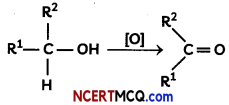= chromium-based reagent, activated DMSO, hypervalent iodide compound, TRAP or TEMPO
R1 = alkyl or aryl substituent
R2 = hydrogen, alkyl or aryl substituent
(A) Name the reagent which can be used to oxidise an alcohol to a ketone.
(B) Write the aldehyde and the ketone formed by the oxidation of propan-l-ol and propan-2-ol respectively.
(C) Propanal can be oxidized easily than propanone. Give reason.
(D) Give simple chemical tests to distinguish between the following pairs of compounds.
(i) Propanal and Propanone
(ii) Ethanal and Propanal
OR
How will you prepare the following compounds starting with benzene:
(i) Benzaldehyde
(ii) Acetophenone (5)
(A) CrO3 with heat

Caution
If a student writes PCC in place of CrO3, it will be a wrong answer because PCC (mild oxidizing agent) can be used to oxidise a primary alcohol to an aldehyde.

(B) Proparial and Propanone

Explanation:
Propan-1-ol being a primary alcohol on oxidation gives propanal an oldehyde and Propan-2-ol being a secondary alcohol on oxidation gives propanone.

(C) The difference between an aldehyde and ketone is the presence of a hydrogen atom attached to the carbon-oxygen double bond in the aldehyde. Ketone doesn’t have that hydrogen attached. Due to electron-withdrawing nature of C=O group, C—H bond in aldehyde is weak and thus it can be easily oxidised to corresponding Corboxylic acid.

(D) (i) Propanal. (aldehyde) and propanone (ketone):
Propanol gives sâ.er mirror test with Totten’s reagent. Propanone does not gives this test.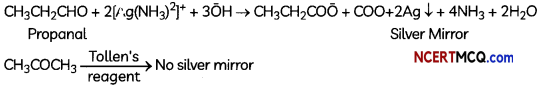(ii) Ethonal and proponat can be distinguished by Iodoforrn test. Ethanal gives a yellow precipitate of iodofirm with an aLkaline so4ution of NaOH. Propanal does not gives this test.